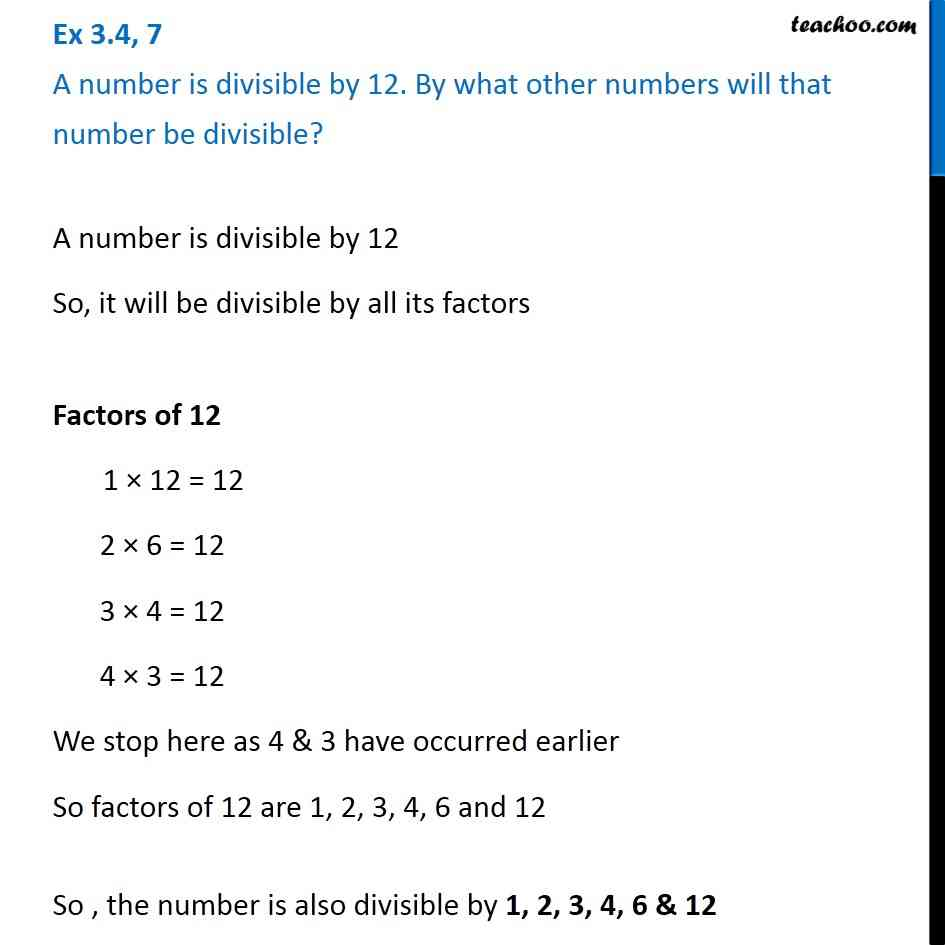Ex 3.4

Chapter 3 Class 6 Playing with Numbers
Serial order wiseLearn in your speed, with individual attention - Teachoo Maths 1-on-1 Class

### Transcript

Ex 3.4, 7 A number is divisible by 12. By what other numbers will that number be divisible? A number is divisible by 12 So, it will be divisible by all its factors Factors of 12 1 × 12 = 12 2 × 6 = 12 3 × 4 = 12 4 × 3 = 12 We stop here as 4 & 3 have occurred earlier So factors of 12 are 1, 2, 3, 4, 6 and 12 So , the number is also divisible by 1, 2, 3, 4, 6 & 12